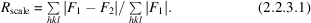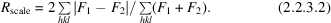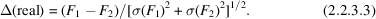International
Tables for
Crystallography
Volume F
Crystallography of biological macromolecules
Edited by E. Arnold, D. M. Himmel and M. G. Rossmann

International Tables for Crystallography (2012). Vol. F, ch. 2.2, p. 68

## Section 2.2.3. Comparing different diffraction data sets

H. M. Einspahra* and M. S. Weissb

aPO Box 6483, Lawrenceville, NJ 08648–0483, United States, and bHelmholtz-Zentrum Berlin für Materialien und Energie, Macromolecular Crystallography (HZB-MX), Albert-Einstein-Str. 15, D-12489 Berlin, Germany
Correspondence e-mail:  hmeinspahr@yahoo.com

### 2.2.3. Comparing different diffraction data sets

| top | pdf |

In MX, there are many instances when two or more diffraction data sets have to be compared to each other. An important decision which has to be made is, for instance, whether data sets collected from different crystals are isomorphous enough so that they can be merged together. Another task is the comparison of a native data set and a heavy-atom derivative. The question here is how much of the observed difference is due to non-isomorphism and how much is due to isomorphous replacement.

Scaling R factor, Rscale. The scaling R factor Rscale between two data sets is defined as the difference in the structure-factor amplitudes of one data set relative to the structure-factor amplitudes of the other data set after the two data sets have been scaled to each other.However, the above formula is asymmetric with respect to data sets 1 and 2. An alternative symmetric formula isRscale is also often given based on intensities rather than structure-factor amplitudes.

Gradient from a normal probability analysis. For each reflection hkl in two data sets that have been scaled to each other, the quantity Δ(real) is computed and compared with its expectation value Δ(expected) assuming a normal distribution of errors (Howell & Smith, 1992). If Δ(real) is plotted against Δ(expected), random differences between the two data sets give rise to a slope of 1.0. Significant differences result in slopes significantly larger than 1.0. Such an analysis is implemented in the program SCALEIT (Collaborative Computational Project, Number 4, 1994).### References

Collaborative Computational Project, Number 4 (1994). The CCP4 suite: programs for protein crystallography. Acta Cryst. D50, 760–763.
Howell, P. L. & Smith, G. D. (1992). Identification of heavy-atom derivatives by normal probability methods. J. Appl. Cryst. 25, 81–86.